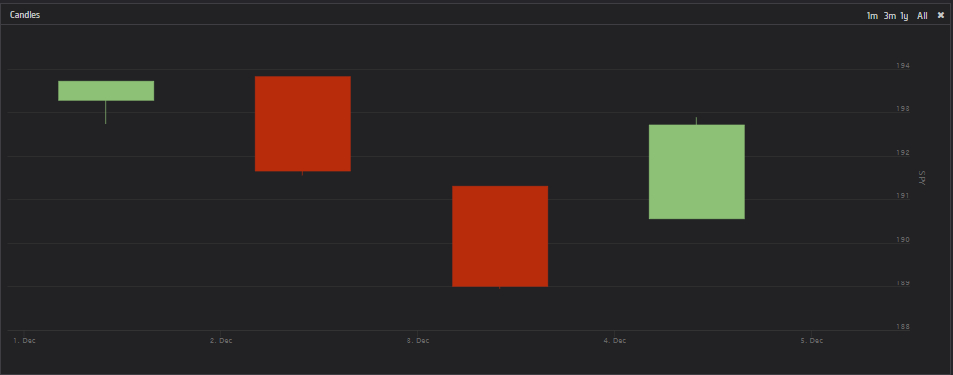I want to plot 60 minutes candles for SPY using the following code:

```### <summary> ### Simple multi-fimeframe forex scalping based on https://www.youtube.com/watch?v=zhEukjCzXwM ### </summary>> class Plotting(QCAlgorithm):     def Initialize(self):         '''Initialise the data and resolution required, as well as the cash and start-end dates for your algorithm. All algorithms must initialized.'''         self.SetStartDate(2015, 12, 1)    #Set Start Date         self.SetEndDate(2015, 12, 5)      #Set End Date         self.SetCash(5000)             #Set Strategy Cash         self.forexPair = "EURUSD"         self.AddEquity('SPY', Resolution.Hour)                         chart = Chart("Candles")         price = Series("SPY", SeriesType.Candle)         chart.AddSeries(price)         self.AddChart(chart)                          def OnData(self,data):         self.Plot("Candles","SPY", self.Securities['SPY'].Close)```

But instead of one candle each 60 minutes, I get one candle per day:What am I doing wrong?